Courses

# Test: Cumulative Frequency Curve

## 15 Questions MCQ Test Mathematics (Maths) Class 10 | Test: Cumulative Frequency Curve

Description
This mock test of Test: Cumulative Frequency Curve for Class 10 helps you for every Class 10 entrance exam. This contains 15 Multiple Choice Questions for Class 10 Test: Cumulative Frequency Curve (mcq) to study with solutions a complete question bank. The solved questions answers in this Test: Cumulative Frequency Curve quiz give you a good mix of easy questions and tough questions. Class 10 students definitely take this Test: Cumulative Frequency Curve exercise for a better result in the exam. You can find other Test: Cumulative Frequency Curve extra questions, long questions & short questions for Class 10 on EduRev as well by searching above.
QUESTION: 1

### The following table gives the marks obtained by 80 students in a selection test: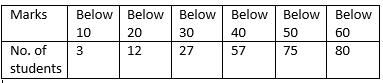The median marks is​

Solution:

Median =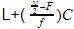First change the table into continuous data.
Middle of the frequencies=40 which will lie in 30-40. So
L=30
N/2=80/2=40
F=27
f=30
c=10
Median =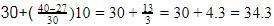QUESTION: 2

Solution:
QUESTION: 3

### Modal class of the following distribution is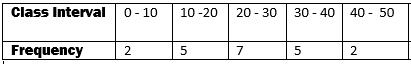​

Solution:
QUESTION: 4

The median of a distribution divides it into​

Solution:
QUESTION: 5

In a shooting competition the scores of a competitor were as given below: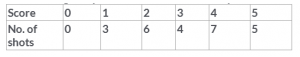His median score will be

Solution:
QUESTION: 6

In a continuous distribution with usual notations, if l = 32.5, f= 15, f= 12, f= 8 and size of each class is 8, then the mode of data is​

Solution:
QUESTION: 7

The arithmetic mean of the following data is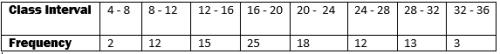Solution:
QUESTION: 8

The measure of central tendency that is most suitable to find the average male or female height of the region is

Solution:

Mean also called the expected value or average, is the central value of a discrete set of numbers. Median is the middle value of the data. Mode is the highest occurring observation. So mean is accurate to calculate average.

QUESTION: 9

The mean and the median of a distribution are 45.9 and 46 respectively. The mode will be​

Solution:
QUESTION: 10

Mode is not affected by​

Solution:

The mode of a set of data values is the value that appears most often . Since mode is the most occurrence of observation, it doesn’t get affected either by minimum value or maximum value or by both the extreme values.

QUESTION: 11

For the following distribution the difference in the upper limit of median and modal class is​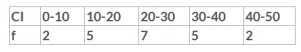Solution:
QUESTION: 12

If there are two class intervals 10-20 and 20-30, then in which interval will 20 fall?​

Solution:
QUESTION: 13

The following table gives the marks obtained by 80 students in a selection test: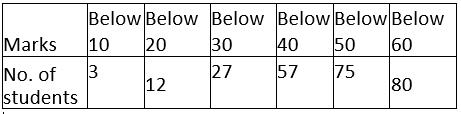The median marks is​

Solution:
QUESTION: 14

For the following distribution the difference in the upper limit of median and modal class is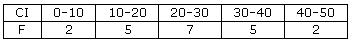Solution:
QUESTION: 15

The mean of 15 numbers is 25. If each number is multiplied by 4, mean of the new numbers is​

Solution:

We have So, Mean =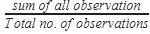So Mean =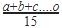=25
If 4 is multiplied by each observation
So Mean =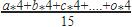Taking common 4 from numerator=4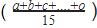=4*25=100